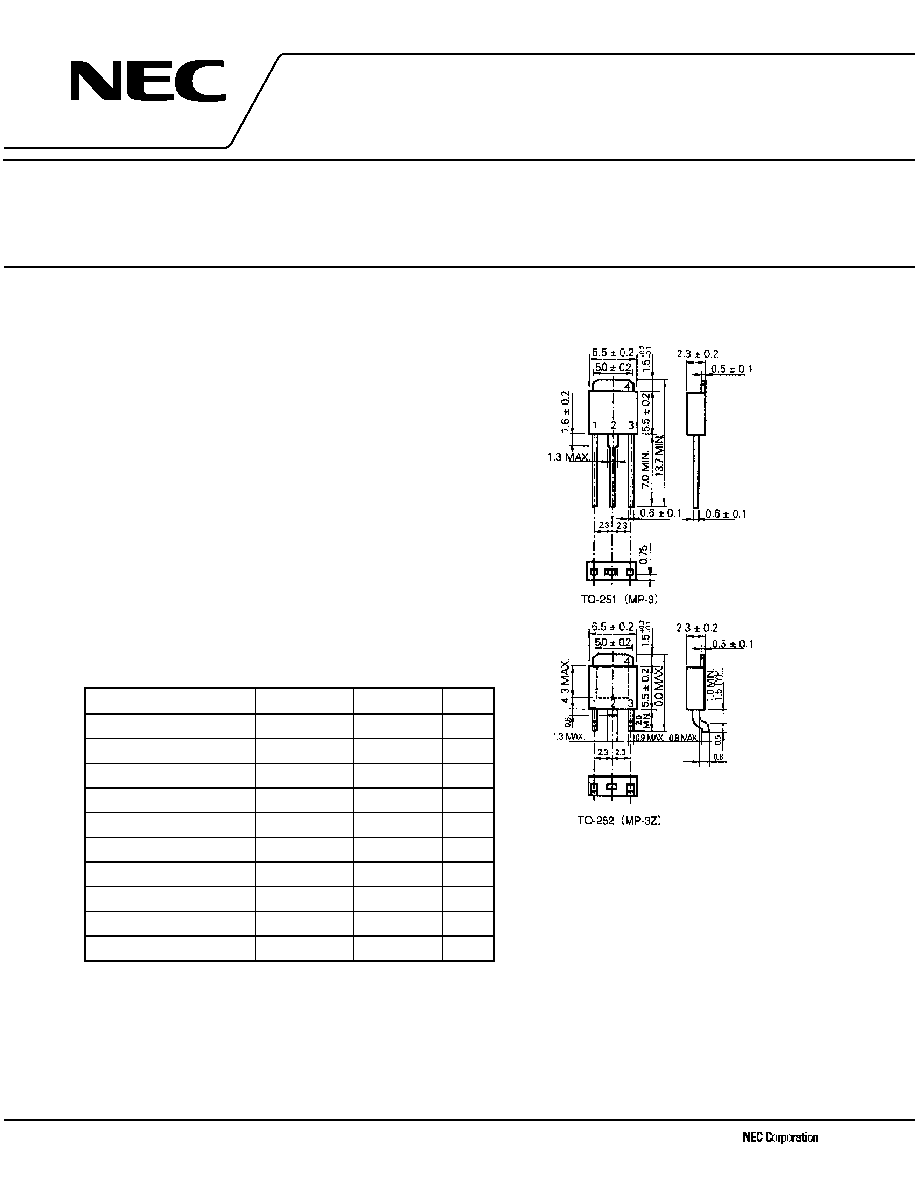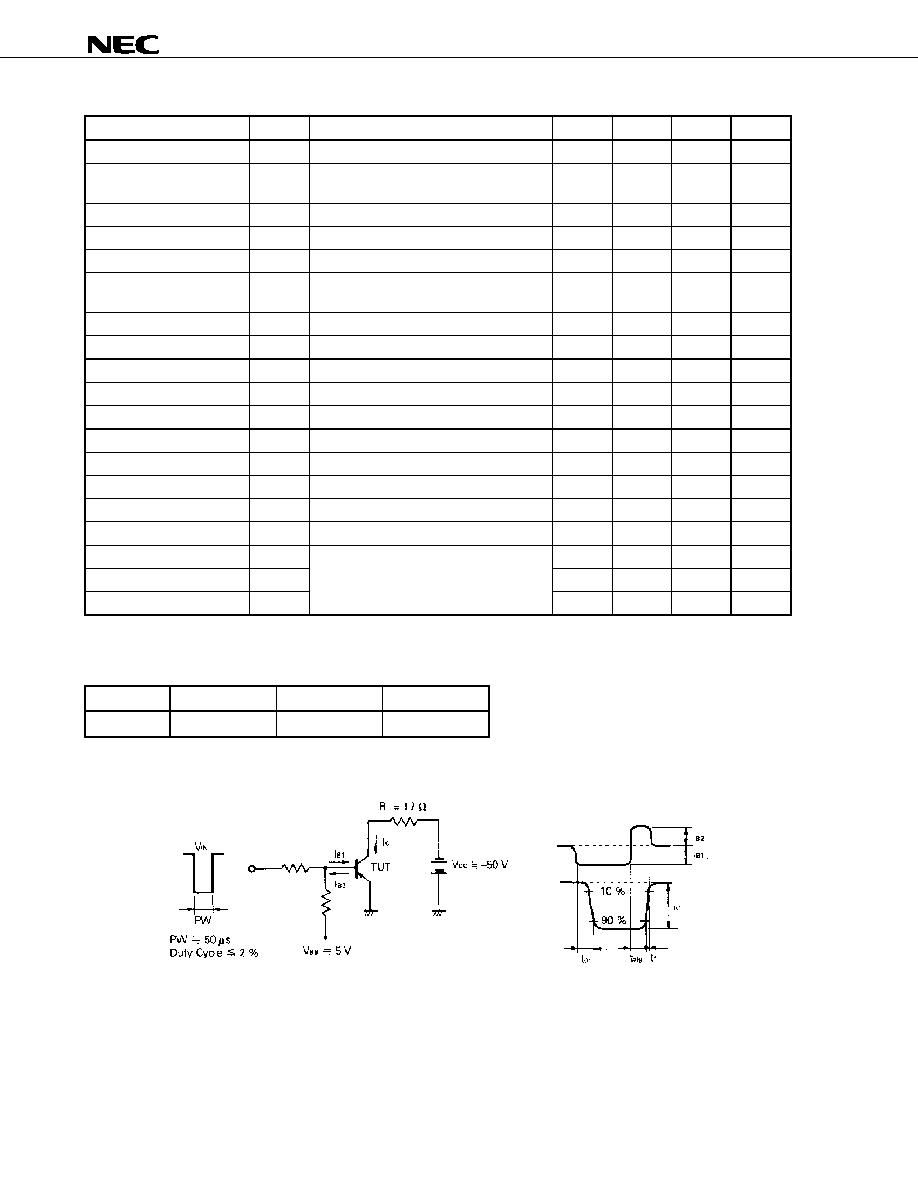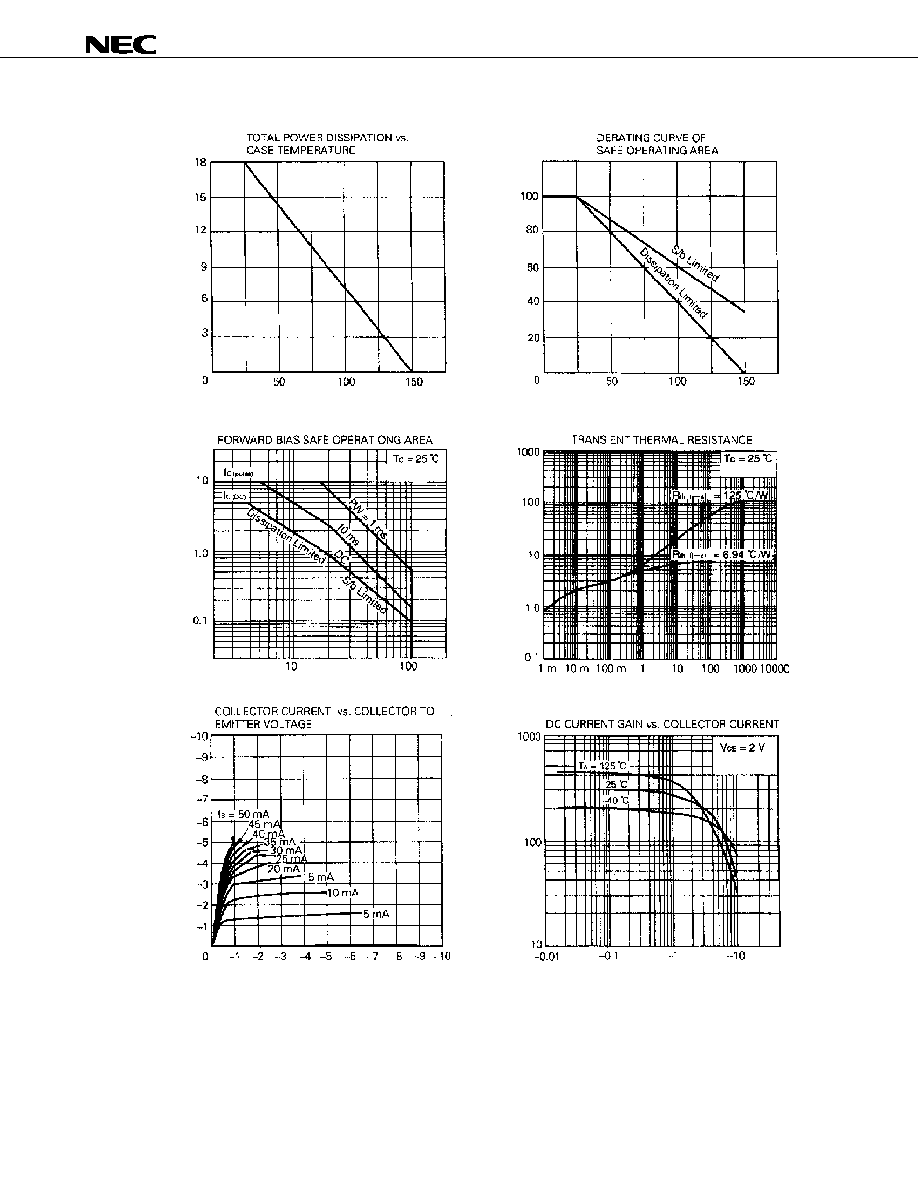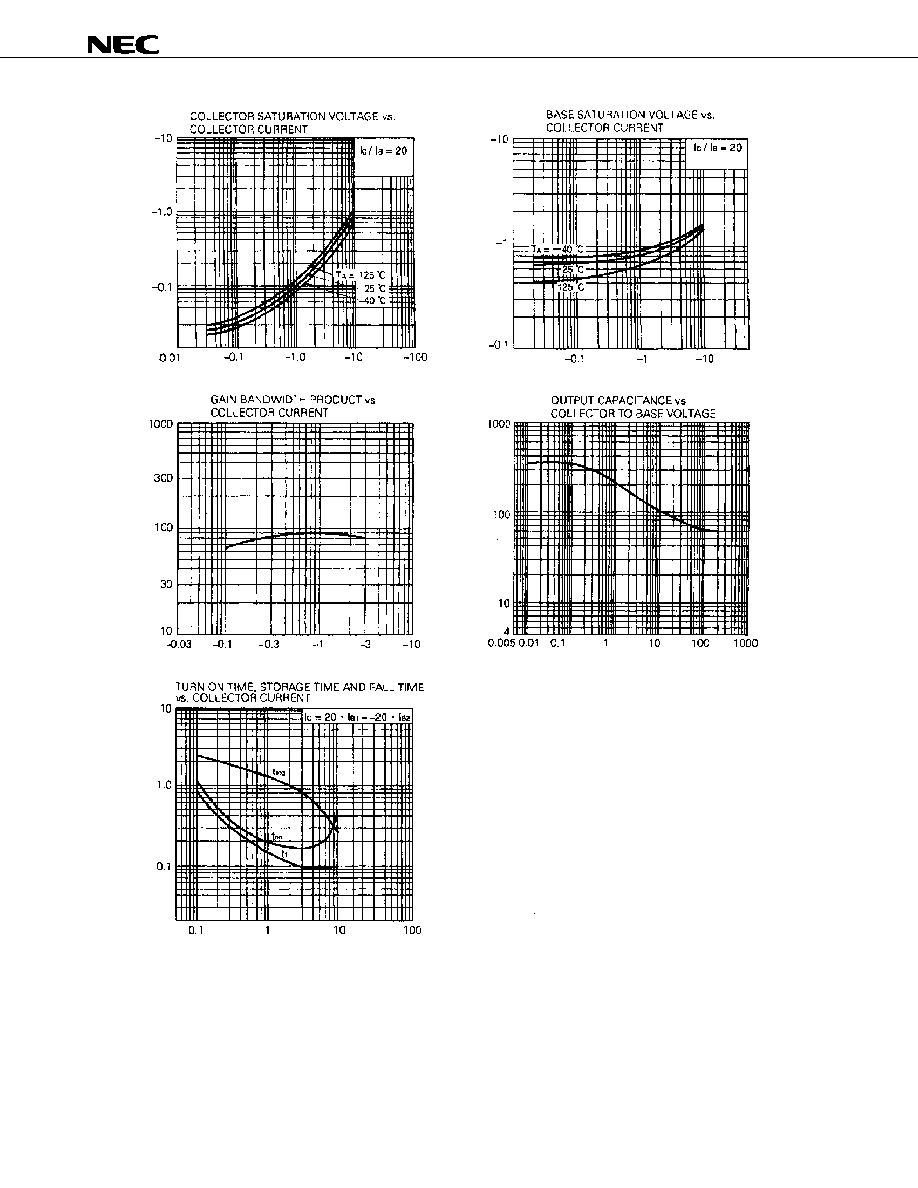Поиск даташита (1.687.043 компонентов) Где искатьв наименованиив описании### Datasheet: 2SA1647 (NEC)

Silicon Power Transistor
Скачать:PDFZIP# Document Outline

• COVER'RFXPHQW 1R '(-9'6 QG HGLWLRQ
'DWH 3XEOLVKHG \$SULO 1 &3.
3ULQWHG LQ -DSDQ
6,/,&21 32:(5 75\$16,6725
6\$ 6\$=
313 6,/,&21 (3,7\$;,\$/ 75\$16,6725
)25 +,*+63((' 6:,7&+,1*
'\$7\$ 6+((7
The information in this document is subject to change without notice. Before using this document, please
confirm that this is the latest version.
Not all devices/types available in every country. Please check with local NEC representative for
availability and additional information.
2002
The 2SA1647 is a mold power transistor developed for high-
speed switching and features a very low collector-to-emitter
saturation voltage.
This transistor is ideal for use in switching regulators, DC/DC
converters, motor drivers, solenoid drivers, and other low-voltage
power supply devices, as well as for high-current switching.
FEATURES
� Available for high-current control in small dimension
� Z type is a lead processed product and is deal for mounting a
hybrid IC.
� Low collector saturation voltage:
V
CE(sat)
=
-0.3 V MAX. (@I
C
=
-3 A)
� Fast switching speed:
t
f
= 0.4
�s MAX. (@I
C
=
-3 A)
� High DC current gain and excellent linearity
ABSOLUTE MAXIMUM RATINGS (T
A
= 25

C)
Parameter
Symbol
Ratings
Unit
Collector to base voltage
V
CBO
-150
V
Collector to emitter voltage
V
CEO
-100
V
Base to emitter voltage
V
EBO
-7.0
V
Collector current (DC)
I
C(DC)
-5.0
A
Collector current (pulse)
I
C(pulse)
*
-10
A
Base current (DC)
I
B(DC)
-2.5
A
Total power dissipation
P
T
(Tc = 25
�C)
18
W
Total power dissipation
P
T
(T
A
= 25
�C)
1.0**, 2.0***
W
Junction temperature
T
j
150
�C
Storage temperature
T
stg
-55 to +150
�C
PACKAGE DRAWING (UNIT: mm)
(OHFWURGH &RQQHFWLRQ
%DVH
&ROOHFWRU
(PLWWHU
*:
PW
10 ms, duty cycle 50%
**: Printing board mounted
***: 7.5 mm
� 0.7 mm ceramic board mountedData Sheet D14839EJ2V0DDS
2
2SA1647, 2SA1647-Z
ELECTRICAL CHARACTERISTICS (T
A
= 25

C)
Parameter
Symbol
Conditions
MIN.
TYP.
MAX.
Unit
Collector to emitter voltage
V
CEO(SUS)
I
C
=
-2.5 A, I
B
=
-0.25 A, L = 1 mH
-100
V
Collector to emitter voltage
V
CEX(SUS)
I
C
=
-2.5 A, I
B1
=
-I
B2
=
-0.25 A,
V
BE(OFF)
= 1.5 V, L = 180
�H, clamped
-100
V
Collector cutoff current
I
CBO
V
CB
=
-100 V, I
E
= 0
-10
�A
Collector cutoff current
I
CER
V
CE
=
-100 V, R
BE
= 50
, T
A
= 125
�C
-1.0
mA
Collector cutoff current
I
CEX1
V
CE
=
-100 V, V
BE(OFF)
= 1.5 V
-10
�A
Collector cutoff current
I
CEX2
V
CE
=
-100 V, V
BE(OFF)
= 1.5 V,
T
A
= 125
�C
-1.0
mA
Emitter cutoff current
I
EBO
V
EB(OFF)
=
-5.0 V, I
C
= 0
-10
�A
DC current gain
h
FE1
*
V
CE
=
-2.0 V, I
C
=
-0.5 A
100
DC current gain
h
FE2
*
V
CE
=
-2.0 V, I
C
=
-1.0 A
100
400
DC current gain
h
FE3
*
V
CE
=
-2.0 V, I
C
=
-3.0 A
60
Collector saturation voltage
V
CE(sat)1
*
I
C
=
-3.0 A, I
B
=
-0.15 A
-0.3
V
Collector saturation voltage
V
CE(sat)2
*
I
C
=
-4.0 A, I
B
=
-0.2 A
-0.5
V
Base saturation voltage
V
BE(sat)1
*
I
C
=
-3.0 A, I
B
=
-0.15 A
-1.2
V
Base saturation voltage
V
BE(sat)2
*
I
C
=
-4.0 A, I
B
=
-0.2 A
-1.5
V
Collector capacitance
C
ob
V
CB
=
-10 V, I
E
= 0, f = 1.0 MHz
110
pF
Gain bandwidth product
f
T
V
CE
=
-10 V, I
C
= 0.5 A
90
MHz
Turn-on time
t
on
0.3
�s
Storage time
t
stg
1.5
�s
Fall time
t
f
I
C
=
-3.0 A, R
L
= 17
,
I
B1
=
-I
B2
=
-0.15 A, V
CC
-50 V
Refer to the test circuit.
0.4
�s
* Pulse test PW
350
�s, duty cycle 2%/Pulsed
h
FE
CLASSIFICATION
Marking
M
L
K
h
FE2
100 to 200
150 to 300
200 to 400
SWITCHING TIME TEST CIRCUIT
Base current
waveform
Collector current
waveformData Sheet D14839EJ2V0DDS
3
2SA1647, 2SA1647-Z
TYPICAL CHARACTERISTICS (T
A
= 25

C)
T
o
t
a
l
P
o
w
e
r Di
ss
i
pat
i
o
n

P
T
(W
)
Case Temperature T
C
(
�C)
I
C

Derat
i
ng
dT
(%
)
Case Temperature T
C
(
�C)
Trans
i
ent
Ther
m
a
l
Res
i
s
t
an
c
e

r
th
(
j
-
c)

(t
)
(

C/
W
)
Pulse Width PW (s)
Collector to Emitter Voltage V
CE
(V)
Collector to Emitter Voltage V
CE
(V)
Col
l
e
c
t
or Current

I
C
(A
)
Col
l
e
c
t
or Current

I
C
(A
)
Single pulse
Single pulse
Collector Current I
C
(A)
DC Current
Gai
n

h
FE
Pulse testData Sheet D14839EJ2V0DDS
4
2SA1647, 2SA1647-Z
Collector Current I
C
(A)
Collector Current I
C
(A)
Collector Current I
C
(A)
C
o
l
l
ec
t
o
r
S
a
t
u
r
a
t
i
o
n
V
o
l
t
ag
e V
CE
(
s
a
t
)
(V)
G
a
i
n
B
a
n
d
w
i
dt
h
P
r
od
u
c
t
f
T
Tur
n
-
O
n
Ti
m
e
t
on
(
s)
S
t
or
ag
eTi
m
e
t
st
g
(
s)
Fal
l
T
i
m
e

t
f
(
s)
Pulse test
Collector to Base Voltage V
CB
(V)
C
o
l
l
ec
t
o
r
C
a
pac
i
t
a
n
c
e

C
ob
(p
F)
Collector Current I
C
(A)
Ba
s
e
S
a
t
u
r
a
ti
o
n

Vo
lta
g
e
V
BE(s
a
t
)
(V)
Pulse testData Sheet D14839EJ2V0DDS
5
2SA1647, 2SA1647-Z
[MEMO]© 2019 • ChipFind• Контакты• Главная страница